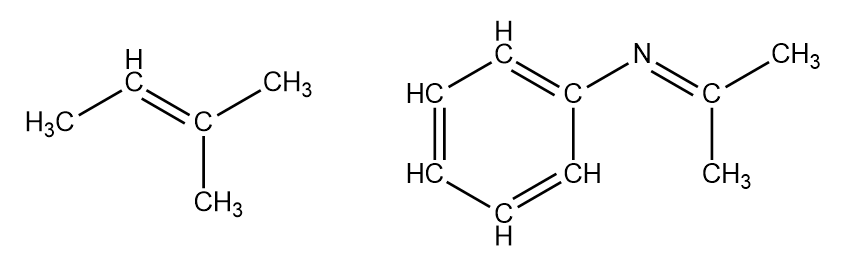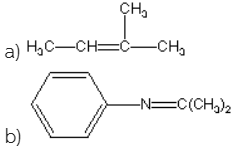# Problem: 1) How many kinds of chemically non-equivalent hydrogens are there in each of the following compounds?

###### FREE Expert Solution

For this problem, we need to determine how many kinds of chemically non-equivalent Hs in each of the ff. compounds• Recall that for 1H-NMR analysis, there are as many signals on each spectrum as there are unique, non-equivalent protons
• In this case, let's assume that Hs bound to the same C is equivalent
• Take note that symmetry will reduce the total number of signals
100% (73 ratings)###### Problem Details

1) How many kinds of chemically non-equivalent hydrogens are there in each of the following compounds?